# 分类模型的F1分值、Precision和Recall 计算过程

## 引入

accuracy = (分类正确的样本个数) / (分类的所有样本个数)

## 二分类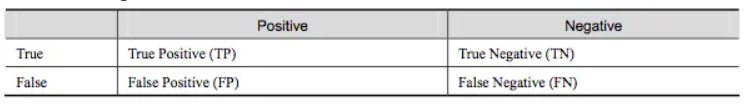• TP：预测为Positive并且对了（样本为正类且预测为正类）
• TN：预测为Negative并且对了（样本为负类且预测为负类）
• FP：预测为Positive但错了（样本为负类但预测为正类）
• FN：预测为Negative但错了（样本为正类但预测为负类）
• TP+FP：预测为Positive并且对了+预测为Positive但错了=预测为Positive的样本总数
• TP+FN：预测为Positive并且对了+预测为Negative但错了=实际为Positive的样本总数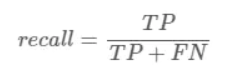F1是调和平均值，精准率和召回率只要有一个比较小的话，F1的值也会被拉下来：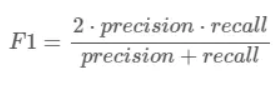## 多分类情况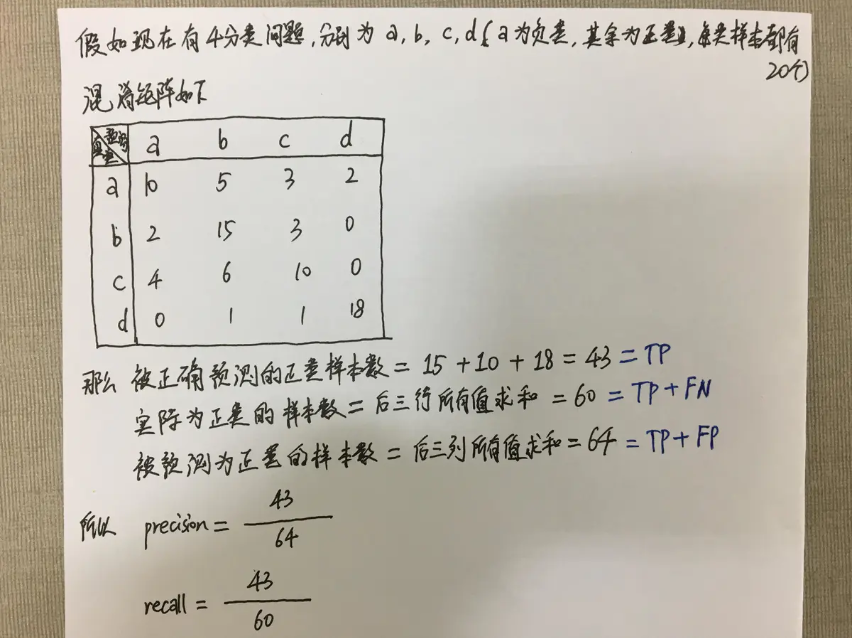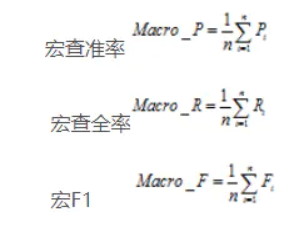Macro情况下上述例子的计算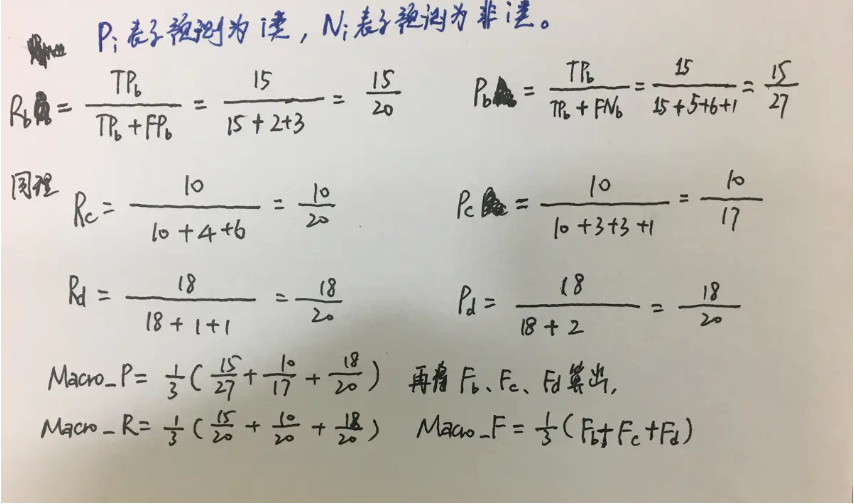## sklearn计算程序（macro）

``````from sklearn.metrics import f1_score, precision_score, recall_score

y_true=[1,2,3]
y_pred=[1,1,3]

f1 = f1_score( y_true, y_pred, average='macro' )
p = precision_score(y_true, y_pred, average='macro')
r = recall_score(y_true, y_pred, average='macro')

print(f1, p, r)
# output: 0.555555555556 0.5 0.666666666667
``````

https://blog.csdn.net/ybdesire/article/details/96507733

https://www.jianshu.com/p/14b26f59040b